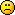<![CDATA[Math Is Fun Forum / Probability function]]> 2018-12-23T15:07:09Z FluxBB https://www.mathisfunforum.com/viewtopic.php?id=24693 <![CDATA[Re: Probability function]]> hi Spicca

Welcome to the forum.

I've been hoping that someone would post an answer to this and I could learn too.  No such luckSo I'm having a go myself.  Please note: I've never done this before, so what follows may be rubbish.  Please comment …. ask for clarification  … tell me why it's wrong etc.  Maybe between us, we can arrive at the correct answer.  And oh yes … your English is good.

So let's work with 3D coordinates with the x-y plane horizontal and z going straight up.  Further, let's make the sphere have unit radius (cannot see any harm in that) and centred on the origin, O.

If P is one point of the tetrahedron, then we can specify its position using spherical coordinates theta and phi as shown here: https://en.wikipedia.org/wiki/Spherical … ate_system  And let P' be the point in the x-y plane below P.

Now, what would be helpful is to have a formula for the volume of a tetrahedron in terms of theta and phi but I cannot find one.  Plenty of internet pages giving the formula in vector terms such as https://math.stackexchange.com/question … ot-product

and as a determinant https://stackoverflow.com/questions/986 … n-4-points

I could also expand either into a large algebraic formula but it would take ages to enter all the LaTex so you'll have to ask nicelyif you want this.

phi can take any random value from 0 to 2pi and theta any from 0 to pi.

So you can construct your function with 8 variables and there it is.  Hhhmmmm.Bob

]]>
https://www.mathisfunforum.com/profile.php?id=67694 2018-12-23T15:07:09Z https://www.mathisfunforum.com/viewtopic.php?pid=406556#p406556
<![CDATA[Re: Probability function]]> For  the  problem  of  2-dimensional  , let  O  be  the  centre  of  the  circle  . Take  a  point  A  on  the  circumference  ,  and  let  AD  be  the  diameter  with  length  2  unit . If  we  take  2  more  points  B  and  C  randomly  on  the  circumference  to  form  a  triangle ,  the  chance  that  B  and  C  lie  on  the  same  side  of  AD  is  1/2 ,  while  the  chance  that  B  and  C  lie  on  the  opposite  sides  of  AD  is  also  1/2 . We  shall  consider  the  1st  case  firstly . Let  x  be  the  length  of  AB , and  let  y  be  the  length  of  AC . ( For  simplicity  let  x ≤ y ) Then  both  x  and  y  varies  from  0  to  2  units .
We  know  that  the  area  of  Δ ABC  =  Δ ABO  +  Δ OBC  -  Δ ACO .  But  how  to  find  the  areas  of  the  last  3   Δ s  ?

]]>
https://www.mathisfunforum.com/profile.php?id=212022 2018-12-23T07:01:27Z https://www.mathisfunforum.com/viewtopic.php?pid=406549#p406549
<![CDATA[Re: Probability function]]> Hi  Spicca ,

I  really  cannot  solve  this  problem . Or  we  may  consider  a  similar  but  simpler  one  of  2- dimensional  ,  perhaps  we  can  get  some  hint .

From  a  circle  with  radius  1  unit , 3  points  are  picked  randomly  on  its  circumference . Find  the  expected  area  of  the  triangle  so  formed .

]]>
https://www.mathisfunforum.com/profile.php?id=212022 2018-12-11T04:45:29Z https://www.mathisfunforum.com/viewtopic.php?pid=406412#p406412
<![CDATA[Probability function]]> Pick 4 random points on a sphere. what Probability function has the volume of tetrahedron with these points?

I've seen the question of average volume from somewhere and I am interested in the probabilities of the volume. (unfortunately I couldn't understand the answer...)

I'm sorry if my english is bad. If you don't understand anything please let me know. Thank you!

]]>
https://www.mathisfunforum.com/profile.php?id=219728 2018-12-05T09:10:55Z https://www.mathisfunforum.com/viewtopic.php?pid=406359#p406359